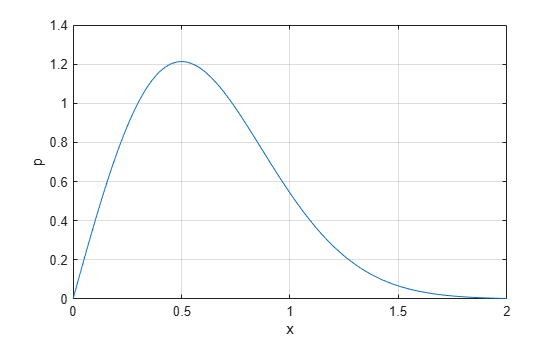# raylpdf

Rayleigh probability density function

## Syntax

```Y = raylpdf(X,B) ```

## Description

`Y = raylpdf(X,B)` computes the Rayleigh pdf at each of the values in `X` using the corresponding scale parameter, `B`. `X` and `B` can be vectors, matrices, or multidimensional arrays that all have the same size, which is also the size of `Y`. A scalar input for `X` or `B` is expanded to a constant array with the same dimensions as the other input.

The Rayleigh pdf is

`$y=f\left(x|b\right)=\frac{x}{{b}^{2}}{e}^{\left(\frac{-{x}^{2}}{2{b}^{2}}\right)}$`

## Examples

collapse all

Compute the pdf of a Rayleigh distribution with parameter `B = 0.5`.

```x = [0:0.01:2]; p = raylpdf(x,0.5);```

Plot the pdf.

```figure; plot(x,p)```